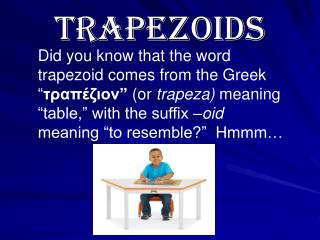# Trapezoids - PowerPoint PPT PresentationDownload PresentationTrapezoids

TrapezoidsDownload Presentation## Trapezoids

- - - - - - - - - - - - - - - - - - - - - - - - - - - E N D - - - - - - - - - - - - - - - - - - - - - - - - - - -
##### Presentation Transcript

1. Did you know that the word trapezoid comes from the Greek “τραπέζιον” (or trapeza) meaning “table,” with the suffix –oid meaning “to resemble?” Hmmm… Trapezoids

2. Definition of trapezoid… • Trapezoid: a quadrilateral consisting of exactlyonepair of parallel sides. OR a quadrilateral with two parallel sides of unequal length. • Using this definition, is a square also a trapezoid? A rectangle? A parallelogram?

3. Trapezoids: b1 = base 1 h = height leg leg Height – distance between the 2 bases. * Must be  b2 = base 2 base base Area of trapezoid A = ½ h(b1 + b2) Height

4. Ex. 1 Find the area of the following trapezoid. 20in This is the height!! 30in 18in 36in A = ½ h(b1 + b2) = ½ (18in)(36in + 20in) = ½ (18in)(56in) = 504in2

5. What happens if you are given the area, but are missing one of the bases? = 4 in b1 4 in A = 22 in2 7 in

6. Find the height of the trapezoid. 35 m = 23 m h A = 1035 m2 55 m